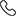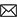Sales :9214233303 / Tech. Support Desk :9214233360/40 (9AM ~ 6PM)# Foundation Coordinate Geometry ,Linear Equation in Two Variable for class 9th by MC Sir

The Cartesian plane, coordinates of a point, names and terms associated with the coordinate plane, notations, plotting points in the plane.

Recall of linear equations in one variable. Introduction to the equation in two variables. Focus on linear equations of the type ax+by+c=0. Prove that a linear equationin two variables has infinitely many solutions and justify their being written as ordered pairs of real numbers, plotting them and showing that they seem to lie on a line. Graph of linear equations in two variables.

• Rs.1,000 Rs.1,000
• Rs.2,000 Rs.2,000
• Rs.1,000 Rs.1,000
Subject Medium Target Language Mathematics English IX Class English + Hindi Topic Based 11 Videos 0 Videos PDF Files Full coverage of topics Coordinate Geometry & Linear Equation in Two Variables 9th class Properly organized solutions in chapter-wise and page wise format help find your solution quickly Must for class 8th and 9th studying students who want to do well in NTSE, Olympiad, KVPY, IIT JEE, Medical and other engineering and competitive exams Ideal for class 9th students who are preparing for NTSE, Olympiad, KVPY, IIT JEE Exams. Essential for higher mathematics learning students. Essential for CBSE, NIOS & ICSE Exam preparations

### Other Courses by Manoj Chauhan SirTap a Star to Rate us:
close
close